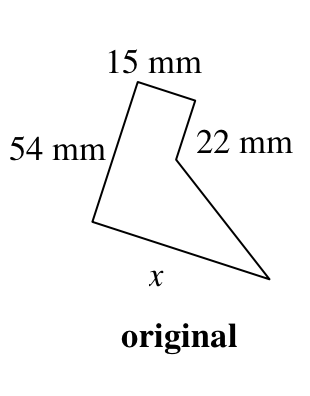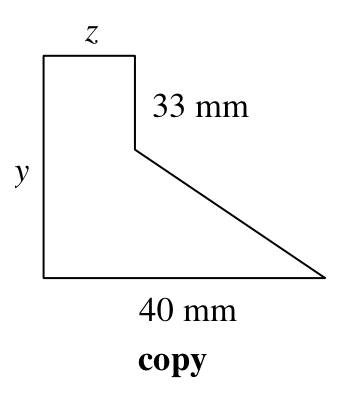### Home > CC2MN > Chapter 5 > Lesson 5.2.4 > Problem5-72

5-72.

Find the lengths of the missing sides on the similar shapes at right. What is the scale factor?

Similar shapes have corresponding sides with equal ratios to each other.

To find each unknown side, use the ratio between two known corresponding sides.

For example, the ratio of the $15$ mm side to the $22$ mm side should be equal to the ratio of the $z$ side to the $33$ mm side.

$\frac{15}{22} = \frac{z}{33}$
Now solve for $z$.

$z=22.5$ mm
You can now use that to find ratios for the other unknown sides.

Write two more ratios to solve for side $x$ and side $y$.

$\frac{15}{54} = \frac{22.5}{y}$
$15y=(22.5)(54)$

$y=81$ mm
Now what does $x$ equal?